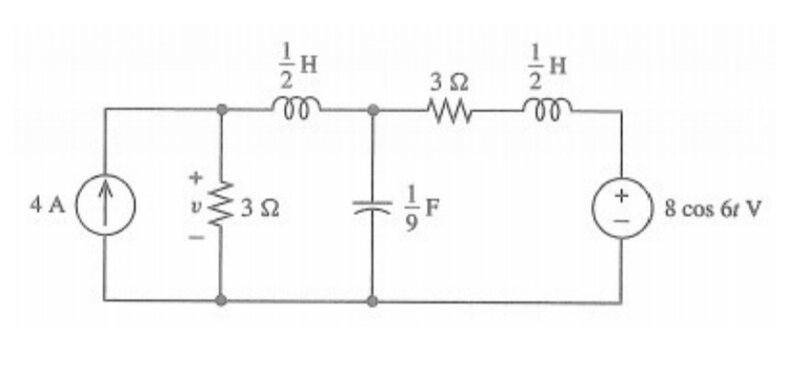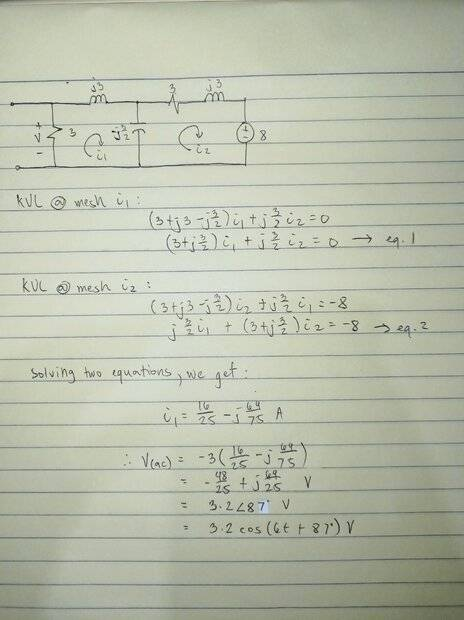# How to deal with dc source on ac circuits?

• Engineering
• Secre

#### Secre

Homework Statement
Find the steady state voltage v.
Relevant Equations
v(t) = V(dc) + V(ac)We are tasked to solve for the steady state voltage v using superposition theorem. I start with solving with voltage source using mesh analysis and got the current flowing through the 3 ohms resistor and get the first value of the voltage which is 3.2cos(6t+126.87). Now my problem is I am confused on how to deal with the other source since we're in ac analysis. I tried solving it with dc analysis but I think it's wrong.

Have you started using Phasors yet or analyzing AC circuits with a single fixed frequency?

Yes

Great! The 4A current source looks like a/an __________ for AC, so you can probably use the Phasor impedances to write the AC KCL equation(s)...

Should I assume w= 0 rad/s for the current source since I'll be needing it to convert it into phasor circuit?

Well, I'd just ignore the DC current source for the AC analysis, since an ideal current source has infinite output resistance (looks like an open circuit). Can you write the Phasor KCL equation for the top middle node (since the DC current source is not an open)?To get the contribution to v from the 4 amp source just start by short circuiting the voltage source. Now in the steady state the inductors with be short circuits and the capacitor will be an open circuit. You are then left with three parallel circuit elements (one current source and two 4 ohm resistors.) I think you can take it from there.